## Fisher's Exact Test

A Statistical Test used to determine if there are nonrandom associations between two Categorical Variables. Let there exist two such variables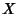and, withandobserved states, respectively. Now form an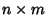Matrix in which the entriesrepresent the number of observations in whichand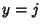. Calculate the row and column sums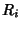and, respectively, and the total sumof the Matrix. Then calculate the conditional Likelihood (P-Value) of getting the actual matrix given the particular row and column sums, given by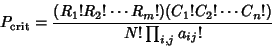(which is a Hypergeometric Distribution). Now find all possible Matrices of Nonnegative Integers consistent with the row and column sumsand. For each one, calculate the associated P-Value using (0) (where the sum of these probabilities must be 1). Then the P-Value of the test is given by the sum of all P-Value which are.

The test is most commonly applied to aMatrices, and is computationally unwieldy for largeor.

For an example application of the test, letbe a journal, say either Mathematics Magazine or Science, and letbe the number of articles on the topics of mathematics and biology appearing in a given issue of one of these journals. If Mathematics Magazine has five articles on math and one on biology, and Science has none on math and four on biology, then the relevant matrix would be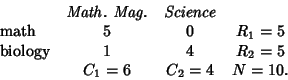Computinggivesand the other possible matrices and their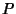s are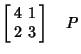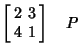which indeed sum to 1, as required. The sum of-values less than or equal tois then 0.0476 which, because it is less than 0.05, is Significant. Therefore, in this case, there would be a statistically significant association between the journal and type of article appearing.